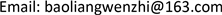1. 引言

2. 特征分析

2.1. 轴特征在工程图中的形状表示

2.2. 特征轮廓环之间的关系

1) 相邻关系

2) 包含关系

3) 切入关系

3. 条件随机场(CRFs)模型

3.1. 条件随机场定义

3.2. 条件随机场概率模型的形式

p ( y | x ) = 1 Z ( x ) ∏ t = 1 T exp { ∑ k = 1 K     λ k f k ( y t , y t ` , x t ) }

4. 构造轴的训练模型4.1. 构造无向图

4.2. 特征集

1) 边界线/非边界线，位于视图边界的轮廓线被定义为边界线否则，这是一条非边界线。

2) 切割线，一条切割线显示了一个物体被切割的位置，以获得剖面图。

Attribute feature set and relational feature se

4.3. 特征函数

p ( y | x ) = 1 Z ( x ) exp { ∑ k ∑ i = 1 n − 1   λ k t k ( y i + 1 , y i , x i ) + ∑ j ∑ i = 1 n   μ j s j ( y i , x i ) }

s j ( y i , x i ) = { 1             如 是 关 于 中 心 线 对 称 0                                 其 它

t k ( y i + 1 , y i , x i ) = { 1         如 是 相 邻 环 0                 其 它

4.4. 参数估计和预测

L ( θ ) = ∑ i = 1 N log p ( y ( i ) | x (i) )

L ( θ ) = ∑ i = 1 N log p ( y ( i ) | x ( i ) ) − ∑ k = 1 K λ k 2 2 ω 2

L ( θ ) λ k = ∑ i = 1 N ∑ t = 1 T   f k ( y t ( i ) , y t ` ( i ) , x t ( i ) ) − ∑ i = 1 N ∑ t = 1 T ∑ y = y ′   f k ( y , y ′ , x t ( i ) ) p ( y , y ′ | x ( i ) ) − ∑ k = 1 K λ k 2 2 ω 2

y * = arg max y p ( y | x )

5. 实现和识别测试

5.1. 样本标记和训练

1) 对工程图纸进行分析，标记轴零件工程视图上轮廓环

2) 构造数据训练集

The feature recognition results of the axis in <xref ref-type="fig" rid="fig">Figure </xref>

l1倒角
l2圆柱面
l3
l4退刀槽
l5圆柱面
l6圆柱面
l7键槽
l8倒角

The test results of partial instance recognitio

1l1：倒角；l2：圆柱面；l3：圆柱面； l4：圆柱面；l5：圆柱面；l6：倒角
2l1：倒角；l2：圆柱面；l3：孔； l4：倒角；l5：圆柱面； l6：键槽；l7：倒角
3l1：倒角；l2：圆柱面；l3：键槽；l4：圆柱面； l5：圆柱面；l6：圆柱面；l7：键槽；l8：倒角

3) 通过收集的数据训练集训练每个特征的特征函数及其环与环之间的转移特征函数，训练出参数 θ = { λ k } 。

5.2. 识别测试

6. 结论